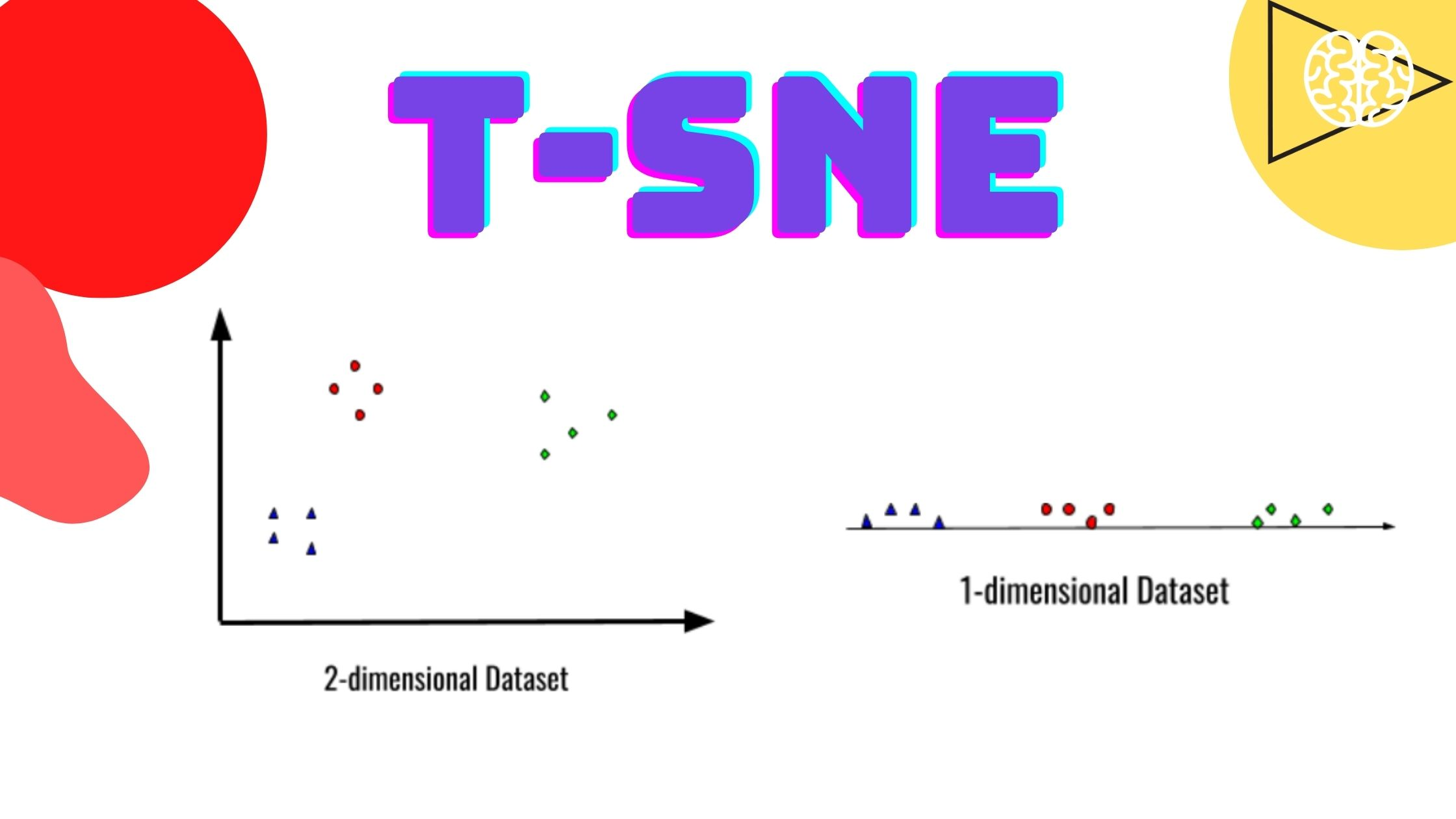# What is t-SNE? Is it Better than PCA?

## Introduction

The dataset with higher dimensionality has always been a problem for all the machine learning algorithms, it becomes very difficult for a machine learning or deep learning algorithm to understand the dataset and extract some useful information out of it for further prediction if the dimensions are high.

Moreover, high dimensional dataset tends to be unstructured and can become really nasty with all those noises. Data mining also becomes hard due to such noise in a dataset. If someone is confused about what is high dimensional data and what is low dimensional data, for them, a high dimensional dataset involves hundreds of features or samples whereas a low dimensional dataset contains a few features and samples, also, to find the pattern or relationship among hundreds of features is itself a huge task which is why we use dimensionality reduction technique.

## Dimensionality Reduction

Dimensionality reduction is a technique with which we lower the dimensions or the number of features in order to reduce the dimension from the dataset, the only thing which one should remember while performing dimensionality reduction is that it must not lose any useful information.

A good dimensionality reduction technique will not only lower the dimension but it will make sure that no useful information is harmed in the process. There are various machine learning algorithms that are used for dimensionality reduction such as principal component analysis(PCA), Autoencoder, Random Forest, LDA, and more. Today we are going to discuss T-sne, which works better and faster than all the other dimensionality reduction techniques listed above.

## t-SNE

T-sne is a dimensionality reduction technique that uses ‘T-distribution’ to convert the higher dimensional dataset to a lower dimension. The statistic part of the T-sne is quite complex than other techniques, but the intuition is fairly simple. T-sne generally reduces non-linear dimensions without any loss of important data.

Now let’s see an example of how T-sne will convert a 2-dimensional dataset into 1-d.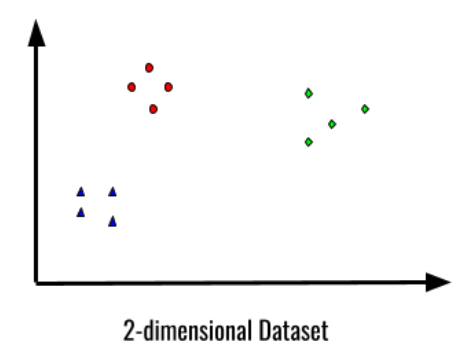So, T-sne can reduce the above two-dimensional dataset into a 1-dimensional dataset, but how could it achieve it without losing any information or how would the above dataset look like in the lower dimension? Let’s see below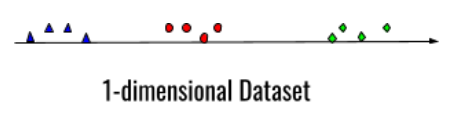This is how the dataset will look after the conversion from 2-dimension to 1-dimension, we can observe the data points that were close to each other are still close to each other and the distance between each cluster is tried to maintain in this dimension too. Also, one more thing to notice here is that all the information is preserved but the dimension has been successfully converted. Now, the question is how does T-sne do it?

## Working of t-SNE

Let us again consider the 2-dimensional data that we have seen above-:Now, if we will directly plot the data in 1-dimension, we will get something like below-: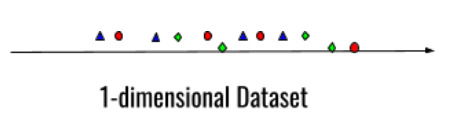We can see that if we just reduce the dimension without using T-sne, this is the result which we will get and this is where T-sne will start its working. Now, if we can see the triangular data point, it wants to get closer to the other triangular data point, similarly, all other data point wants to get closer to their individual clusters.

(Also read: Python Matplotlib for Data Visualization)

## Steps to perform t-SNE

Our first step is to find the similarity of all the points within the scatter plot, to find the similarity we need to find the distance between every data point as that would be the similarity criteria for us.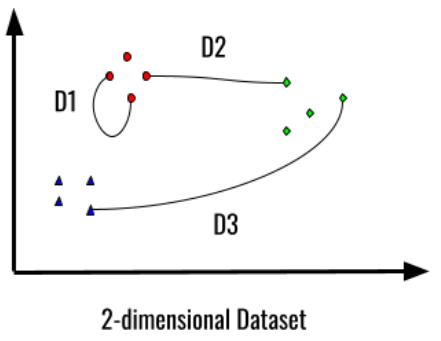Consider the 3 distances that we have taken D1, D2, and D3, we can observe that for D1, the distance between the two points are less than D2 and the distance D2 is less than the distance of D3, therefore, D1 will have high similarity values when we will plot it on a normal curve and D3 will have the lowest similarity value, let’s try to plot it-: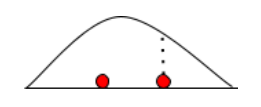Distance D1 on Normal Curve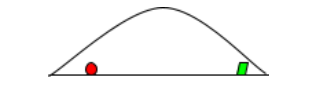Distance D2 on Normal Curve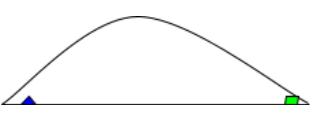Distance D3 on Normal Curve

If you can observe, we can notice that the similarity is high in between two red data points and it makes sense as these two data points belong to the same cluster, if we calculate a similarity score for every data point, based on this similarity score, we can move the datapoints in 1-dimension plot.

With the help of the above example, we can note that the density of the cluster also impacts the similarity between them, for the clusters with less density, the similarity will be less and for the clusters with high density, the similarity will be high. Let’s visualize it -: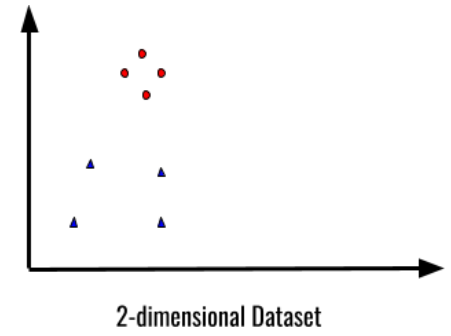There are two clusters in the above 2-dimensional dataset, we can observe red clusters have high density and blue clusters have a low density, if we will plot these two clusters on a normal curve, we will see the difference-: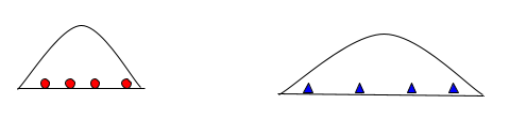The above diagram helps in the visualization of how the density of the clusters impacts the normal curve, but here is one interesting observation, the first normal curve is almost half as compared to the second one, which means that the similarity score will be the same for both the clusters.

“So if the standard deviation for the first curve is 1, and the standard deviation for the second curve is 2, then the similarity score for both the curves are same. “

The formula for scaling similarity score so that they sum to 1 = Score/sum of all score

By calculating the similarity score, we plot the details on the matrice and we can compare it with the original to visualize the similarity between them both. So if we rewind a bit, the steps are-:

Picking a data point on a number line to shift it on its right position, t-SNE doesn’t shift every data point in one iteration, it’s more like sorting algorithms where we try to sort step by step.

Secondly, we need to measure the distance of each and every data point from one another.

Lastly, we need to plot the similarity score using a curve, nowhere is a catch, in the example, we took the normal curve, however, in the t-SNE, we use T-curve to plot the similarity score.

The difference between T-curve and a normal curve is that T-curve is sharper at the top. T-distribution is used in t-SNE to make sure that the clusters don’t stick in the middle, because it becomes harder to see the clusters when they are closely packed.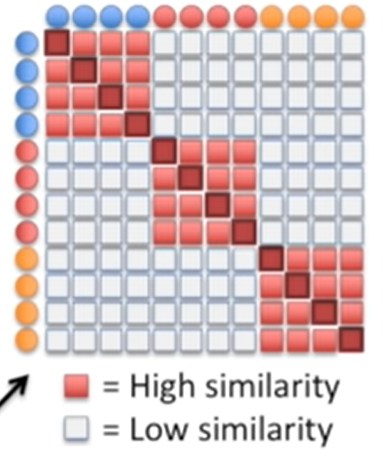Above is a matrix of similarity score, we can visualize that high similarity is denoted with the help of red color, more the similarity, darker will be red color, and if there is white color, then there is no similarity between the two, this is how similarity score helps in finding the right clusters.

Conclusion

To conclude about t-SNE, this dimensionality reduction technique is exceptionally fast, a better optimizer, and handles the outliers better than Principle Component Analysis. Although t-SNE works better than most of the dimensionality reduction techniques, the math behind it is accordingly complex but with the help of the above examples, you might have guessed the intuition behind it.